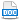HomeTechniques and Tips@RISK DistributionsHow does RiskSplice Work? (Technical Example)

# 3.46. How does RiskSplice Work? (Technical Example)

Applies to: @RISK 5.5.0 and newer

The help text for RiskSplice( ) says

The two pieces of the distribution will be re-weighted since the total area under the (spliced) curve still has to equal 1. Thus the probability density of any given x value in the resulting spliced distribution will probably be different from what it was in the original distribution.

How exactly does RiskSplice( ) work? How are the density functions adjusted to make the new distribution?

Please see the attached Word document for the mathematical details and a complete example.

•SplicedDistributions.docx